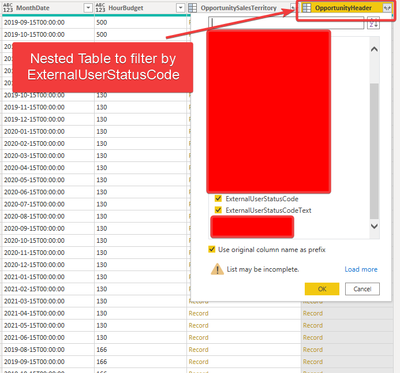cancel
Showing results for
Did you mean:Helper I

## Pre-filtering nested tables before expanding

Hi!

I have an issue where my dataset prior to tranforming is huge. I'm simply trying to filter out the unnecessary data of the nested tables in my odata service prior to expanding the tables.

I can't seem to figure out a solution where I can filter on records, while keeping all of the other data/columns.. is this possible? I tried converting the records to a table and it didn't yield the results I wanted because it gave me a table with only two columns, where all of the column headers from the records table turned into rows with the value and dropped all of the other dataAnd is it possible to filter on more than one value in the same column?

Thanks!!

1 ACCEPTED SOLUTIONSuper User

There's no GUI button but the code looks just like any other filter step.

Here's an example you can paste into the Advanced Editor of a new blank query:

``````let
Source =
#table(
type table [Date = date, Budget = number, Record = record],
{
{#date(2019,09,15), 500, [Code="001", Text="abc", Value=3]},
{#date(2019,10,15), 600, [Code="002", Text="xyz", Value=5]},
{#date(2019,10,31), 400, [Code="003", Text="ijk", Value=8]}
}
),
#"Filtered Rows" = Table.SelectRows(Source, each ([Record][Code] = "003" or [Record][Value] = 5))
in
#"Filtered Rows"``````

Preview:2 REPLIES 2Helper I

This is exactly what I needed and wanted, thank you so much!! Worked perfectlySuper User

There's no GUI button but the code looks just like any other filter step.

Here's an example you can paste into the Advanced Editor of a new blank query:

``````let
Source =
#table(
type table [Date = date, Budget = number, Record = record],
{
{#date(2019,09,15), 500, [Code="001", Text="abc", Value=3]},
{#date(2019,10,15), 600, [Code="002", Text="xyz", Value=5]},
{#date(2019,10,31), 400, [Code="003", Text="ijk", Value=8]}
}
),
#"Filtered Rows" = Table.SelectRows(Source, each ([Record][Code] = "003" or [Record][Value] = 5))
in
#"Filtered Rows"``````

Preview: